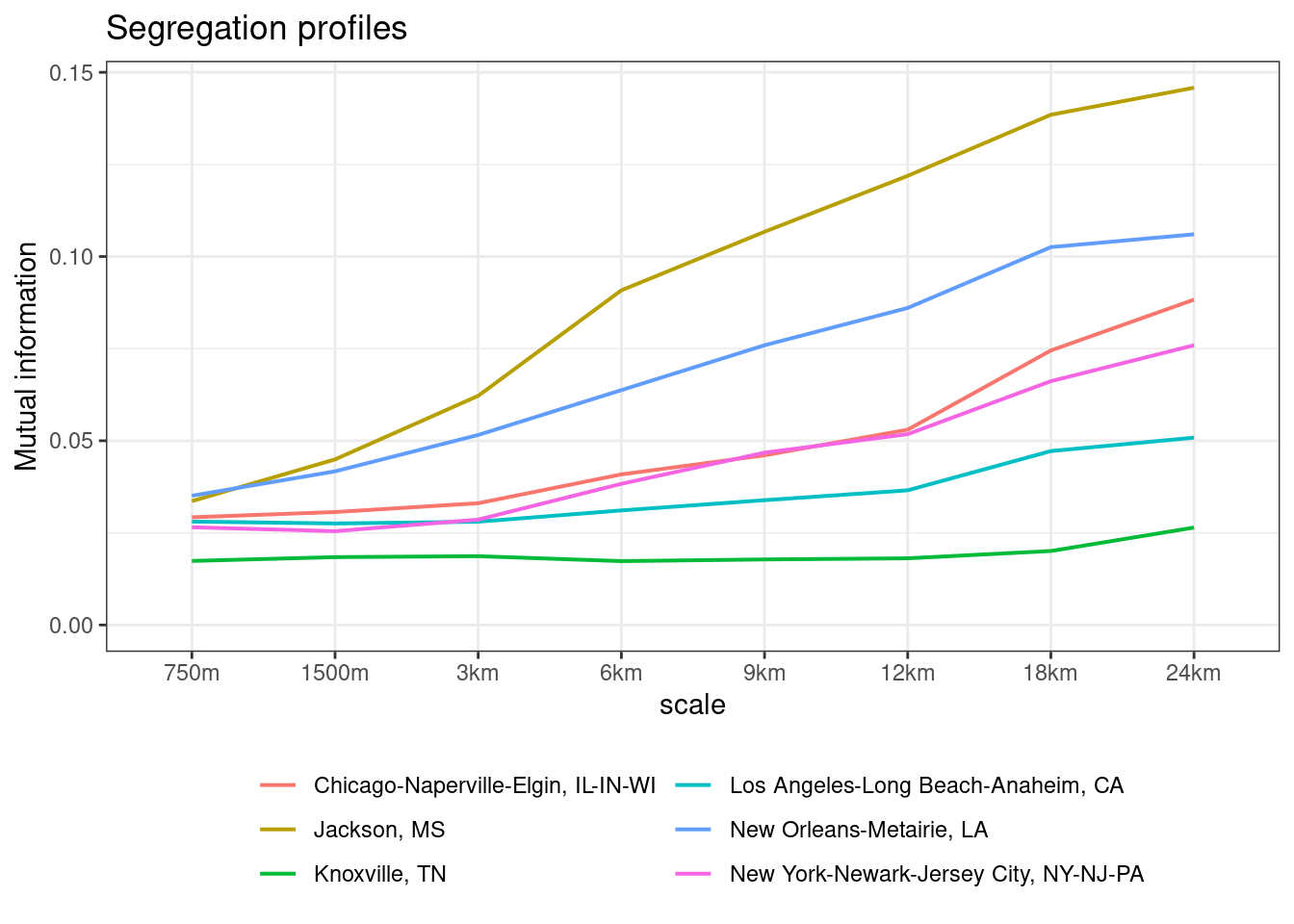Another important inquiry among racial demographers pertains to the appropriate scale at which segregation should be measured. Reardon et al. (2008) introduced the concept of the segregation profile, which represents a function describing the segregation level across different spatial scales. The x-axis shows the scales for which the segregation is calculated, the y-axis is the value of segregation index showing the level of magnitude of segregation.

This example demonstrates the process of obtaining a segregation profile for selected metropolitan statistical areas using the precalculated segregation grids available in the NRGD2020 dataset. The NRGD2020 dataset contains 10 US-wide precalculated layers showing segregation metrics at scales of 72km, 36km, 24km, 18km, 12km, 9km, 6km, 3km, 1.5km, and 0.75km. In segregation grids, the scale is defined by cell size. For example, the result for the scale 3km means that the area was divided into tiles 3x3km, and for each tile, the metrics were calculated. The resulting grid has a resolution of 3x3km.

# 1 Data

The boundaries of the metropolitan statistical areas can be downloaded using tigris package. The example below calculates segregation profiles for 6 metropolitan areas: Chicago IL, New York NY, Los Angeles CA, Knoxville TN, New Orleans LA, Jackson MS. GEOID codes define MSAs for which the calculations are performed.

library(tigris)
library(sf)
#Download CBSA boundaries. Use GEOID code to select metropolitan areas for the analysis.
metro = core_based_statistical_areas(progress_bar = FALSE)
#Transform boundaries to the same crs as grids.
metro = st_transform(metro, crs = 5070)
#Define MSAs included in the analysis by GEOID code. Change this code to calculate the segregation profile for other MSAs. For example, codes 17140, 13820, and 41860 provide segregation profiles for Cincinnati OH, Birmingham AL, and San Francisco CA.
msa_list = metro[metro$GEOID%in%c(16980,31080, 35620, 28940, 35380, 27140),] ## 1.1 Data preparation Data processing consists of a few steps: 1. Download the segregation grids, and the boundary file. 2. Crop US-wide segregation grids to the metropolitan statistical areas. 3. Calculate the mean value of all cells within MSA. It will provide a segregation level for any particular scale. 4. Plot mean segregation values vs scale. # 2 Constructing segregation profile The code below shows how to crop US-wide data within R, calculate mean value of segregation for each scale, and plot the segregation profile. For calculating segregation profiles for selected cities, only their GEOID code is required in the line msa_list = metro[metro$GEOID%in%c(ASSIGN CODE HERE),]. The code below remains unchanged.

library(terra)
library(tidyverse)
library(ggplot2)

#set working directory to the folder containing data using function setwd("")
##setwd("")

#path to US-wide segregation grids
maps = list.files("segregation_grids/", full.names=TRUE)

#scales for calculation in correct order from the smallest to the largest scale
scales = c("750m", "1500m","3km", "6km", "9km", "12km", "18km", "24km")

#crop raster map to the specific boundaries, i.e. MSA and calculate mean value of mutual information
df = data.frame()
for (i in 1:nrow(msa_list)) {
msa = msa_list[i,]

val = numeric()
for (f in maps) {
#read US wide map for particular scale
scale_map = rast(f)
#crop US-wide map to the boundary, i.e MSA
#calculate mean values of metric over all tiles.
v = mean(values(cropped), na.rm=TRUE)
val = c(val, v)
}
#create data.frame with the values of div/seg metric for the different scales for the selected areas (i.e., MSA)
df = rbind(df, val)
}

#process df to use in ggplot library
colnames(df) = sapply(strsplit(basename(maps), "[_. ]+"), function(x) x)
#reorder scales from the smaller to the larger one
df = df[, scales]

#add MSA names to the data.frame
df$msa = msa_list$NAME

#convert from wide to long format
dfl = pivot_longer(df, cols= all_of(scales), names_to = "scale", values_to = "metric")

#Plot segregation profiles
ggplot(dfl, aes(x = factor(scale, level = scales), y = metric, group = msa, color = msa)) +
geom_line(linewidth= 0.7) +
scale_x_discrete(breaks = scales) +
labs(title = "Segregation profiles", x = "scale", y = "Mutual information", color = NULL) +
ylim(0, max(dfl\$metric)) +
theme_bw() + theme(legend.position="bottom")  + guides(color=guide_legend(ncol=2))